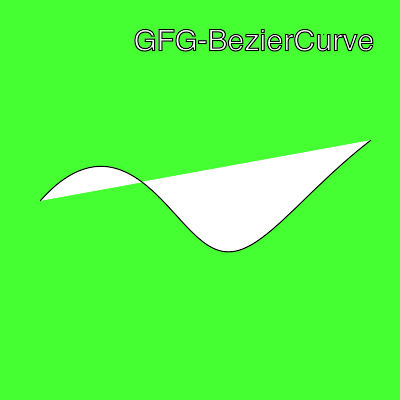# Wand bezier() function – Python

• Last Updated : 08 Jul, 2021

The bezier() function is an inbuilt function in the Python Wand ImageMagick library which is used to draw the bezier curve from specified points.

Syntax:

`bezier(points)`

Parameters: This function accepts single parameter as mentioned above and defined below:

• points: This parameter is used to specify the value of points. It is of List-type which is used to declare array of points.

Return Value: This function returns the Wand ImageMagick object.

Example 1:

## Python3

 `# Import libraries from the wand ``from` `wand.image ``import` `Image``from` `wand.drawing ``import` `Drawing``from` `wand.color ``import` `Color` `with Drawing() as draw:``    ``# Set Stroke color the circle to black``    ``draw.stroke_color ``=` `Color(``'black'``)``    ``# Set Width of the circle to 2``    ``draw.stroke_width ``=` `1``    ``# Set the fill color to 'White (# FFFFFF)'``    ``draw.fill_color ``=` `Color(``'white'``)``    ``# Set the bezier points as (x, y)``    ``points ``=` `[(``40``, ``200``), ``# Start point``    ``(``120``, ``110``), ``# First control``    ``(``190``, ``180``), ``# Second control``    ``(``210``, ``360``), ``# Third control``    ``(``240``, ``240``), ``# Fourth control``    ``(``370``, ``140``)] ``# End point` `    ``# Set the font style``    ``draw.font ``=` `'../Helvetica.ttf'``    ``# Set the font size``    ``draw.font_size ``=` `30``    ` `    ``with Image(width ``=` `400``, height ``=` `400``, background ``=` `Color(``'# 45ff33'``)) as pic:``        ``# Set the text and its location``        ``draw.text(``int``(pic.width ``/` `3``), ``int``(pic.height ``/` `8``), ``'GFG-BezierCurve'``)``        ``# Invoke bezier function with declared points``        ``draw.bezier(points)``        ``# Draw the picture``        ``draw(pic)``        ``# Save the image``        ``pic.save(filename ``=``'bezier1.jpg'``)`

Output:My Personal Notes arrow_drop_up